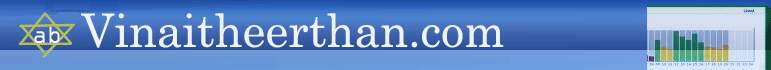Regression

 Name When to use Linear Regression When the dependent variable is conituous and we have one independent variable in a relation Multiple Linear Regression When the dependent vairbale is continuous and we have more than one independent variables in a relation.The Indepedent variables can be continous variables or categoriucal variables It is an extension of Linear Regression Non-Linear Regression When the relationship between the independent and dependent variable assumes non linear relationship, thene non linear regression model is used Two Stage Least Square Regression Two Stage Least Square Regression is used when a predictor variable is correlated with the error term. In the first stage, the variable which is correlated with the error term(endogenous) is estimated from the other variables which are not correlated with the error term(exogenous). In the second stage the estimated variable (exogenous) will be used in the place of the original variable (endogenous). Logistic Regression When the dependent variable is a binary variable and we have one independent variable in a relation Multivariate Logistic Regression When the dependent variable is a binary variable and we have more than one independent variable in a relation.The Indepedent variables can be continous variables or categoriucal variables.It is an extension of Logistic Regression Multinomial Regression When the dependent variable is haveing more than one category  and we have more than one independent variable in a relation Ordinal Regression When the dependent variable is a ordinal varibale  and we have more than one independent variable in a relation Poisson Regression When the dependent variable is a count  varibale  and we have more than one independent variable in a relation Probit Regression Similar to Logistic Regression